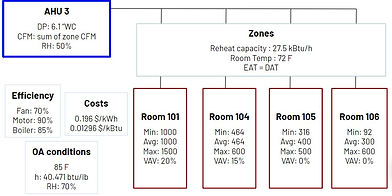top of page## air temperature

Class: Energy Efficient Building Systems (ME 453)

Year: Spring 2019

Goal: To design and conduct an optimization for a system, related to energy efficient building systems

FEATURES

• Custom-made series of programs for the optimization

• Easy user interface, with the user only inputting an excel file with given template

• Conducts operational cost analysis for both each component and overall very quickly

• Visual Representation for clear trends and optimized points

Technical Details

The problem statement for the project was to find the optimal discharge air temperature for Air Handling Unit (AHU) 3, which takes in 100% outside air. This was done by analyzing the power consumption of each component related to the AHU (coil, fan power, reheat), and conducting cost analysis.

The three loads: one for the cooling coil to cool the air, one for the fan to cool the zones (building spaces), and one for the reheat coil in the zones to heat the air to the appropriate Discharge Air Temperature (DAT) if necessary, were analyzed. The possible DAT for this specific system can range from 55 °F to just below 72°F, which is the desired room temperature. The three loads are connected- for example, when the DAT increases, if a zone is in reheat mode, the reheat load should decrease but the load of fan would increase. Thus, the system can be optimized for cost by varying DAT.

Several assumptions were made to analyze the complex system: only the rooms served by the AHU 3 with reheat were considered, and some values, such as outside air conditions and machinery rates, were assumed to be fixed.

The model first calculates the initial conditions based on a given DAT for each zone, and calculating power for the current condition. Then, the model uses these initial conditions and calculates the total loads and operating costs for each possible DAT. The program then plots the values as a visual representation.

To make up for some of the assumptions made in the analysis, the program has several features. For example, the program automatically changes rooms from reheat mode to non-reheat mode if the valve closes, for each DAT. The program also notifies the user when the CFM required for a room is higher than its maximum CFM, and for which DAT it first occurs.

The program has a simple user interface- the user only has to input an excel file in the given format with all the rooms and its specified data, and simply press "run" to execute the program.

All aspects of the optimization was custom-programmed, with an easy-to-use user interface. The technical report can be found here.

bottom of page